Module 4 - Limit as x Approaches a Introduction | Lesson 1 | Lesson 2 | Lesson 3 | Self-Test Lesson 4.3: TI-89 Limit Function The TI-89 computer algebra system has a limit function. In this lesson you will use the TI-89 computer algebra system to find limits. Using the Limit Function From the Home screen, evaluate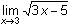by using the limit( command. Display the Calc menu by pressing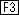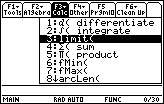Paste the limit( command to the Edit Line by pressing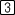Enter the function by pressing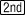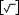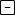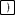Complete and execute the command by pressing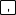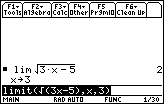So,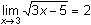. 4.3.1 Use the limit( command to evaluate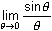. "Sin" is a yellow feature above the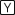key and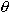is a green feature above the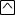key. Be sure the calculator is in radian mode. Click here for the answer. 4.3.2is 1 in Radian mode. Findin Degree mode. Click here for the answer. The Angle mode makes a difference in the evaluation of. Most angle calculations in Calculus are done in Radian mode, so switch back to Radians now. The Sandwich Theorem The Sandwich Theorem is used in many Calculus books to prove= 1. It can be shown that cos<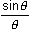< 1 , and because the left and right terms in the inequality approach 1 as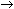0, it must follow that the middle term also approaches 1. This theorem can be visualized by graphing the three terms in the inequality simultaneously. Enter the three functions shown in the Y= menu below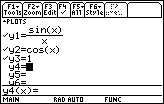The graph format needs to be changed to simultaneous. From the Y= Editor display the Tools menu by pressingHighlight "Format" by using the cursor movement keys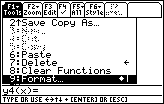Open the Format submenu by pressingDisplay the Graph Order options by pressing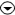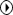Select "SIMUL" by pressingand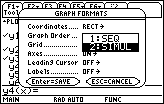Save the Graph Format changes by pressingDisplay graphs of the functions in a [-3, 3] x [-1, 2] window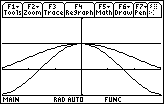Seeing the three graphs simultaneously converge to 1 around x = 0 illustrates the Sandwich Theorem argument that= 1. You can see the three functions converge again by pressing the Regraph button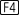. Left- and Right-Hand Limits The limit( command can also be used to evaluate a left-hand limit or a right-hand limit, which is the value approached by a function as x approaches a specific value from the left or from the right. 4.3.3 Interpret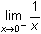and evaluate it with the command limit(1/x,x,0,-1). Click here for the answer. 4.3.4 Interpret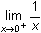and evaluate it by changing –1 to 1 in the Edit Line. Click here for the answer. Using the Trace Feature The Trace feature may be used to estimate limits. As the Trace cursor moves along a curve, the x- and y-values appear at the bottom of the Graph screen. Look at the y-values of y =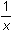as x approaches 0 from the left and from the right by using the Trace feature. Graph y =in a [-3, 3] x [-10, 10] window Activate the Trace cursor by pressingDisplay coordinates of the graph by repeatedly pressingorto move the cursor along the curve Moving the Trace cursor toward zero from the left or the right provides dynamic graphical and numeric reinforcement for the left- and right-hand limits.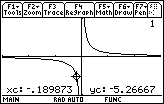Entering a Specific x-Value While the Trace cursor is active, you can move it to a particular point by typing in the x-coordinate of that point. For example, to move to the point with x = –0.001, Enter –0.001. This value should appear by "xc" in the lower left corner of the screen. Execute the command by pressing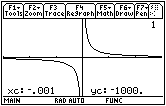The cursor is not visible since it is below the viewing window, but the coordinates of the cursor are shown giving further evidence that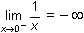. < Back | Next > ©Copyright 2007 All rights reserved. | Trademarks | Privacy Policy | Link Policy